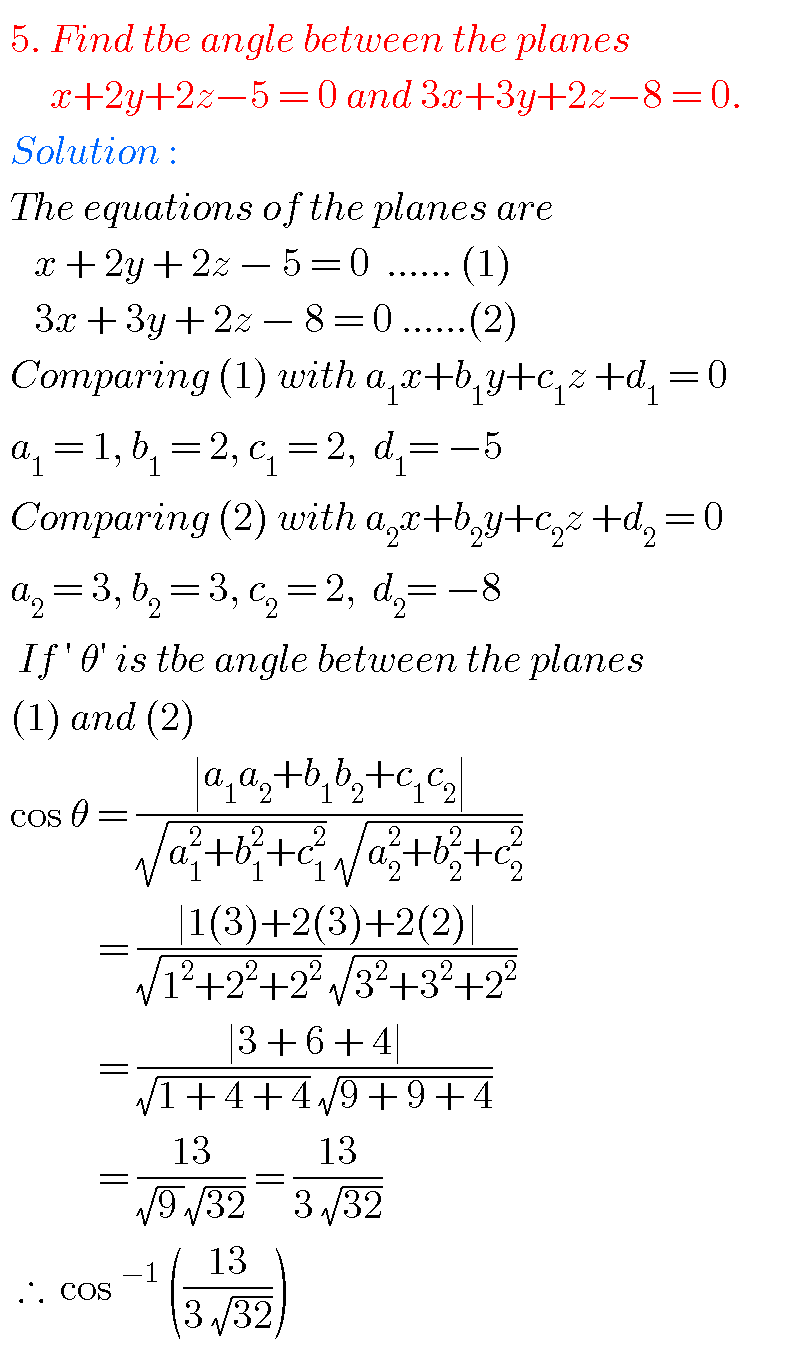## The plane,intermediate first year 1B mathematics chapter 7 problems with solutions,inter maths

THE PLANE, INTERMEDIATE FIRST YEAR MATHEMATICS FIRST YEAR 1B PROBLEMS WITH SOLUTIONS Mathematics intermediate 1a and 1b solutions  for some problems are available here. These are very simple to understand. Inter first year 1a : Functions, mathematical inductions, addition of vectors, product of vectors and trigonometry. Inter first year 1b : Locus, transformation of axes, the straight line, …